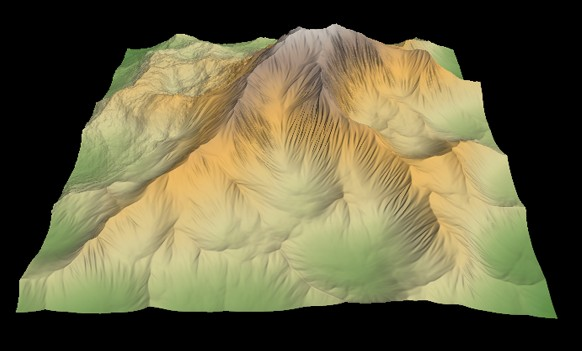### About terrains

Terrains and masks are imported from image files and exported as image files.

What defines a terrain in Instant Terra? A terrain is a square or rectangular grid of a preset size where each vertex of the grid has a width and a height that define a number of squares (quads).

For example, a terrain with a width of 2001 vertices and a height of 1001 vertices has 2001 x 1001 = 2 003 001 vertices, and 2000 x 1000 = 2 000 000 quads.

The length of a quad side determines the total size of the terrain.

For example, for a quad with a length of 1 meter, the terrain has a total size of 2000 meters by 1000 meters. For a quad with a length of 50 cm, the terrain has a total size of 1000 meters by 500 meters.

See the Nodes reference guide for a full list of terrain generation, composition, and transformation nodes.### About masks

Masks are used to specify on which part of a terrain to apply a modification.

Like a terrain, a circular symmetry is a grid that has a width and height in number of vertices and a quad length. Unlike a terrain, each vertex on the grid does not have a height, but a value between 0 (black) and 1 (white).

Masks are imported from an image file or extracted from a terrain. For example, a circular symmetry node may be based on the heights or slopes of a terrain.See the Nodes reference guide for a full list of mask generation, composition, and transformation nodes.

Copyright © 2021 · All Rights Reserved · Wysilab